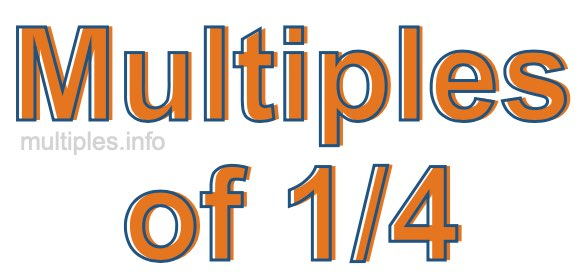Multiples of 1/4We define the multiples of 1/4 as the product of any integer multiplied by 1/4. Therefore, to create a list of multiples of 1/4 we start by multiplying 1/4 by one, then we multiply 1/4 by two, then we multiply 1/4 by three, and so on.

To multiply a fraction by an integer, we leave the denominator alone and multiply the numerator by the integer. It is impossible to give you a list of all multiples of 1/4 because the list goes on forever. However, here is the math to calculate the beginning list of multiples of 1/4.

1/4 × 1 = 1/4

1/4 × 2 = 2/4

1/4 × 4 = 3/4

1/4 × 4 = 4/4

1/4 × 5 = 5/4

1/4 × 6 = 6/4

1/4 × 7 = 7/4

1/4 × 8 = 8/4

1/4 × 9 = 9/4

1/4 × 10 = 10/4

1/4 × 11 = 11/4

1/4 × 12 = 12/4

We have simplified the fractions above for you. Below are the multiples of 1/4 in their lowest possible terms:

1/4 = 1/4

2/4 = 1/2

3/4 = 3/4

4/4 = 1

5/4 = 1 1/4

6/4 = 1 1/2

7/4 = 1 3/4

8/4 = 2

9/4 = 2 1/4

10/4 = 2 1/2

11/4 = 2 3/4

12/4 = 3

As we stated above, the list of multiples of 1/4 goes on forever because the product of 1/4 multiplied by any whole number (integer) is a multiple of 1/4. You can also create a list of multiples of 1/4 if you keep adding 1/4 like this:

1/4
1/4 + 1/4 = 2/4
1/4 + 1/4 + 1/4 = 3/4
1/4 + 1/4 + 1/4 + 1/4 = 4/4

Again, the list can go on forever, because you can continue adding 1/4 to the list. Therefore, the list of multiples of 1/4 is ∞ (infinite).

Multiples of Fractions
Do you need the multiples of another fraction? Enter a another fraction below to get the multiples of that fraction.

/

Multiples of 1/5
Go here for the next fraction on our list that we have multiples for.